# Oxyanion

Updated on
Covid-19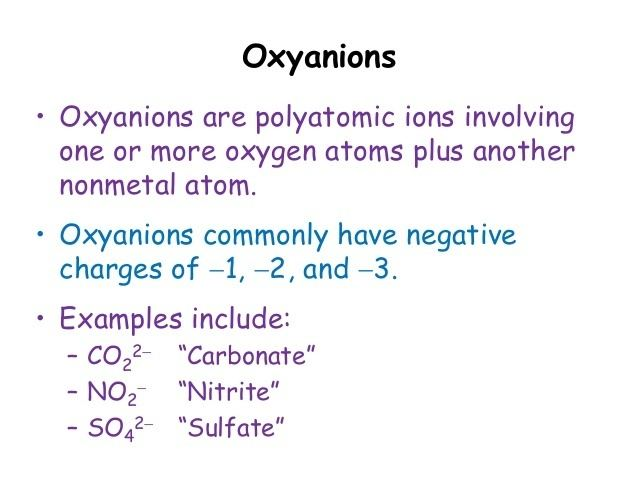## Naming oxyanions

An oxyanion or oxoanion is an ion with the generic formula A
x
Oz
y
(where A represents a chemical element and O represents an oxygen atom). Oxoanions are formed by a large majority of the chemical elements. The formulae of simple oxoanions are determined by the octet rule. The structures of condensed oxoanions can be rationalized in terms of AOn polyhedral units with sharing of corners or edges between polyhedra. The Phosphate and polyphosphate esters adenosine monophosphate (AMP), adenosine diphosphate (ADP) and adenosine triphosphate (ATP) are important in biology.

## Contents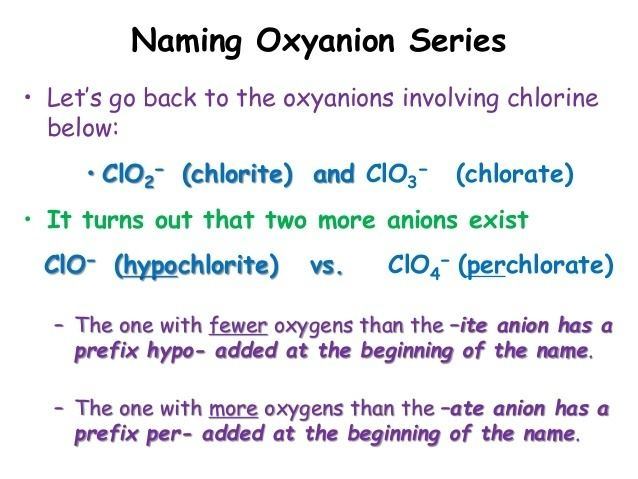## Monomeric oxoanionsThe formula of monomeric oxoanions, AOm
n
, is dictated by the oxidation state of the element A and its position in the periodic table. Elements of the first row are limited to a maximum coordination number of 4. However, none of the first row elements has a monomeric oxoanion with that coordination number. Instead, carbonate (CO2−
3
) and nitrate (NO
3
) have a trigonal planar structure with π bonding between the central atom and the oxygen atoms. This π bonding is favoured by the similarity in size of the central atom and oxygen.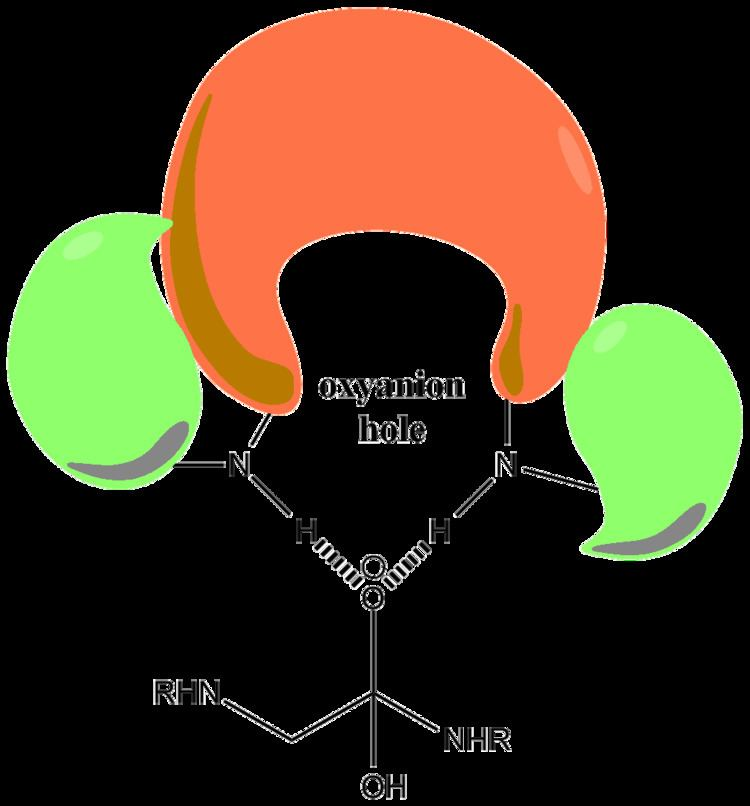The oxoanions of second-row elements in the group oxidation state are tetrahedral. Tetrahedral SiO4 units are found in olivine minerals, [Mg,Fe]SiO4, but the anion does not have a separate existence as the oxygen atoms are surrounded tetrahedrally by cations in the solid state. Phosphate (PO3−
4
), sulfate (SO2−
4
), and perchlorate (ClO
4
) ions can be found as such in various salts. Many oxoanions of elements in lower oxidation state obey the octet rule and this can be used to rationalize the formulae adopted. For example, chlorine(V) has two valence electrons so it can accommodate three electron pairs from bonds with oxide ions. The charge on the ion is +5 − 3 × 2 = −1, and so the formula is ClO
3
. The structure of the ion is predicted by VSEPR theory to be pyramidal, with three bonding electron pairs and one lone pair. In a similar way, the oxyanion of chlorine(III) has the formula ClO
2
, and is bent with two lone pairs and two bonding pairs.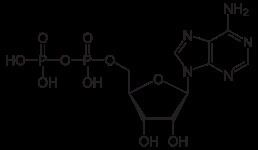In the third and subsequent rows of the periodic table, 6-coordination is possible, but isolated octahedral oxoanions are not known because they would carry too high an electrical charge. Thus molybdenum(VI) does not form MoO6−
6
, but forms the tetrahedral molybdate anion, MoO2−
4
. MoO6 units are found in condensed molybdates. Fully protonated oxoanions with an octahedral structure are found in such species as Sn(OH)2−
6
and Sb(OH)
6
.

## Naming

The naming of monomeric oxoanions follows the following rules.

If central atom is not in Group VII
If central atom is in Group VII

## Condensation reactions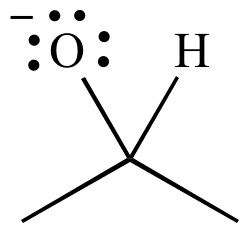In aqueous solution, oxoanions with high charge can undergo condensation reactions, such as in the formation of the dichromate ion, Cr
2
O2−
7
:

2 CrO2−
4
+ 2 H+Cr
2
O2−
7
+ H2O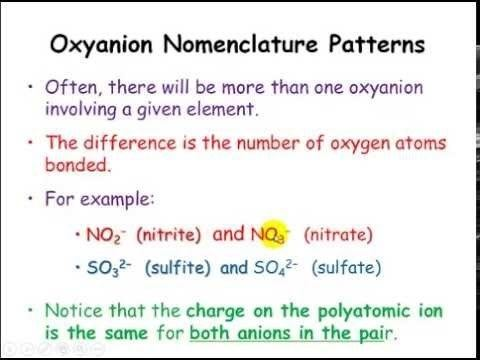The driving force for this reaction is the reduction of electrical charge density on the anion and the elimination of the H+ ion. The amount of order in the solution is decreased, releasing a certain amount of entropy which makes the Gibbs free energy more negative and favors the forward reaction. It is an example of an acid–base reaction with the monomeric oxoanion acting as a base and the condensed oxoanion acting as its conjugate acid. The reverse reaction is a hydrolysis reaction, as a water molecule, acting as a base, is split. Further condensation may occur, particularly with anions of higher charge, as occurs with adenosine phosphates.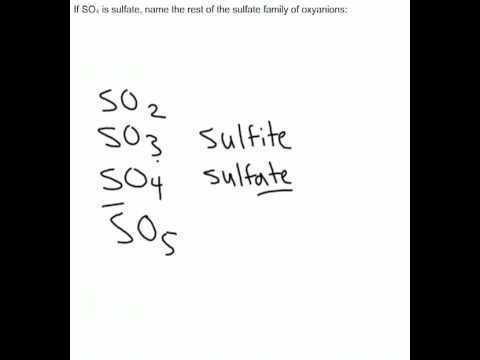The conversion of ATP to ADP is an hydrolysis reaction and is an important source of energy in biological systems.

The formation of most silicate minerals can be viewed as the result of a de-condensation reaction in which silica reacts with a basic oxide, an acid-base reaction in the Lux–Flood sense.

CaO (base) + SiO2 (acid) → CaSiO3

## Structures and formulae of polyoxoanions

A polyoxoanion is a polymeric oxoanion in which multiple oxoanion monomers, usually regarded as MOn polyhedra, are joined by sharing corners or edges. When two corners of a polyhedron are shared the resulting structure may be a chain or a ring. Short chains occur, for example, in polyphosphates. Inosilicates, such as pyroxenes, have a long chain of SiO4 tetrahedra each sharing two corners. The same structure occurs in so-called meta-vanadates, such as ammonium metavanadate, NH4VO3.

The formula of the oxoanion, SiO2−
3
is obtained as follows: each nominal silicon ion (Si4+) is attached to two nominal oxide ions (O2−) and has a half share in two others. Thus the stoichiometry and charge are given by:

Stoichiometry: Si + 2 O + (2 × 12) O = SiO3Charge: +4 + (2 × −2) + (2 × (12 × −2)) = −2.

A ring can be viewed as a chain in which the two ends have been joined. Cyclic triphosphate, P
3
O3−
9
is an example.

When three corners are shared the structure extends into two dimensions. In amphiboles, (of which asbestos is an example) two chains are linked together by sharing of a third corner on alternate places along the chain. This results in an ideal formula Si
4
O6−
11
and a linear chain structure which explains the fibrous nature of these minerals. Sharing of all three corners can result in a sheet structure, as in mica, Si
2
O2−
5
, in which each silicon has one oxygen to itself and a half-share in three others. Crystalline mica can be cleaved into very thin sheets.

The sharing of all four corners of the tetrahedra results in a 3-dimensional structure, such as in quartz. Aluminosilicates are minerals in which some silicon is replaced by aluminium. However, the oxidation state of aluminium is one less than that of silicon, so the replacement must be accompanied by the addition of another cation. The number of possible combinations of such a structure is very large, which is, in part, the reason why there are so many aluminosilicates.

Octahedral MO6 units are common in oxoanions of the larger transition metals. Some compounds, such as salts of the chain-polymeric ion, Mo
2
O2−
7
even contain both tetrahedral and octahedral units. Edge-sharing is common in ions containing octahedral building blocks and the octahedra are usually distorted to reduce the strain at the bridging oxygen atoms. This results in 3-dimensional structures called polyoxometalates. Typical examples occur in the Keggin structure of the phosphomolybdate ion. Edge sharing is an effective means of reducing electrical charge density, as can be seen with the hypothetical condensation reaction involving two octahedra:

2 MOn
6
+ 4 H+Mo
2
O(n−4)−
10
+ 2 H2O

Here, the average charge on each M atom is reduced by 2. The efficacy of edge-sharing is demonstrated by the following reaction, which occurs when an alkaline aqueous solution of molybdate is acidified.

7 MoO2−
4
+ 8 H+Mo
7
O6−
24
+ 4 H2O

The tetrahedral molybdate ion is converted into a cluster of 7 edge-linked octahedra giving an average charge on each molybdenum of 67. The heptamolybdate cluster is so stable that clusters with between 2 and 6 molybdate units have not been detected even though they must be formed as intermediates.

## Heuristic for acidity

The pKa of the related acids can be guessed from the number of double bonds to oxygen. Thus perchloric acid is a very strong acid while hypochlorous acid is very weak. A simple rule usually works to within about 1 pH unit.

## Acid–base properties

Most oxoanions are weak bases and can be protonated to give acids or acid salts. For example, the phosphate ion can be successively protonated to form phosphoric acid.

PO3−
4
+ 3 H+HPO2−
4
+ 2 H+H
2
PO
4
+ H+ ⇌ H3PO4

The extent of protonation in aqueous solution will depend on the acid dissociation constants and pH. For example, AMP (adenosine monophosphate) has a pKa value of 6.21, so at pH 7 it will be about 10% protonated. Charge neutralization is an important factor in these protonation reactions. By contrast, the univalent anions perchlorate and permanganate ions are very difficult to protonate and so the corresponding acids are strong acids.

Although acids such as phosphoric acid are written as H3PO4, the protons are attached to oxygen atoms forming hydroxyl groups, so the formula can also be written as OP(OH)3 to better reflect the structure. Sulfuric acid may be written as O2S(OH)2; this is the molecule observed in the gas phase.

The phosphite ion, PO3−
3
, is a strong base, and so always carries at least one proton. In this case the proton is attached directly to the phosphorus atom with the structure HPO2−
3
. In forming this ion, the phosphite ion is behaving as a Lewis base and donating a pair of electrons to the Lewis acid, H+.

As mentioned above, a condensation reaction is also an acid-base reaction. In many systems, both protonation and condensation reactions can occur. The case of the chromate ion provides a relatively simple example. In the predominance diagram for chromate, shown at the right, pCr stands for the negative logarithm of the chromium concentration and pH stands for the negative logarithm of H+ ion concentration. There are two independent equilibria. Equilibrium constants are defined as follows.

The predominance diagram is interpreted as follows.

• The chromate ion, CrO2−
4
, is the predominant species at high pH. As pH rises the chromate ion becomes ever more predominant, until it is the only species in solutions with pH > 6.75.
• At pH < pK1 the hydrogen chromate ion, HCrO
4
is predominant in dilute solution.
• The dichromate ion, Cr
2
O2−
7
, is predominant in more concentrated solutions, except at high pH.
• The species H2CrO4 and HCr
2
O
7
are not shown as they are formed only at very low pH.

Predominance diagrams can become very complicated when many polymeric species can be formed, such as in vanadates, molybdates, and tungstates. Another complication is that many of the higher polymers are formed extremely slowly, such that equilibrium may not be attained even in months, leading to possible errors in the equilibrium constants and the predominance diagram.

Oxyanion Wikipedia

Topics